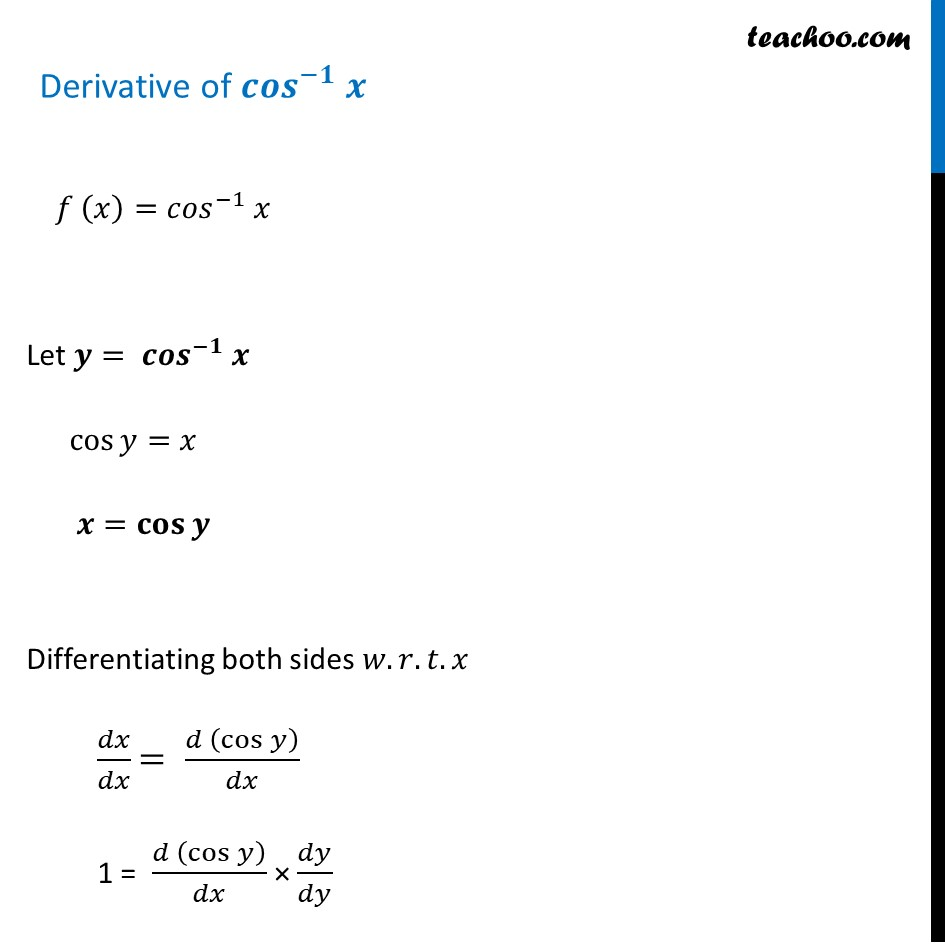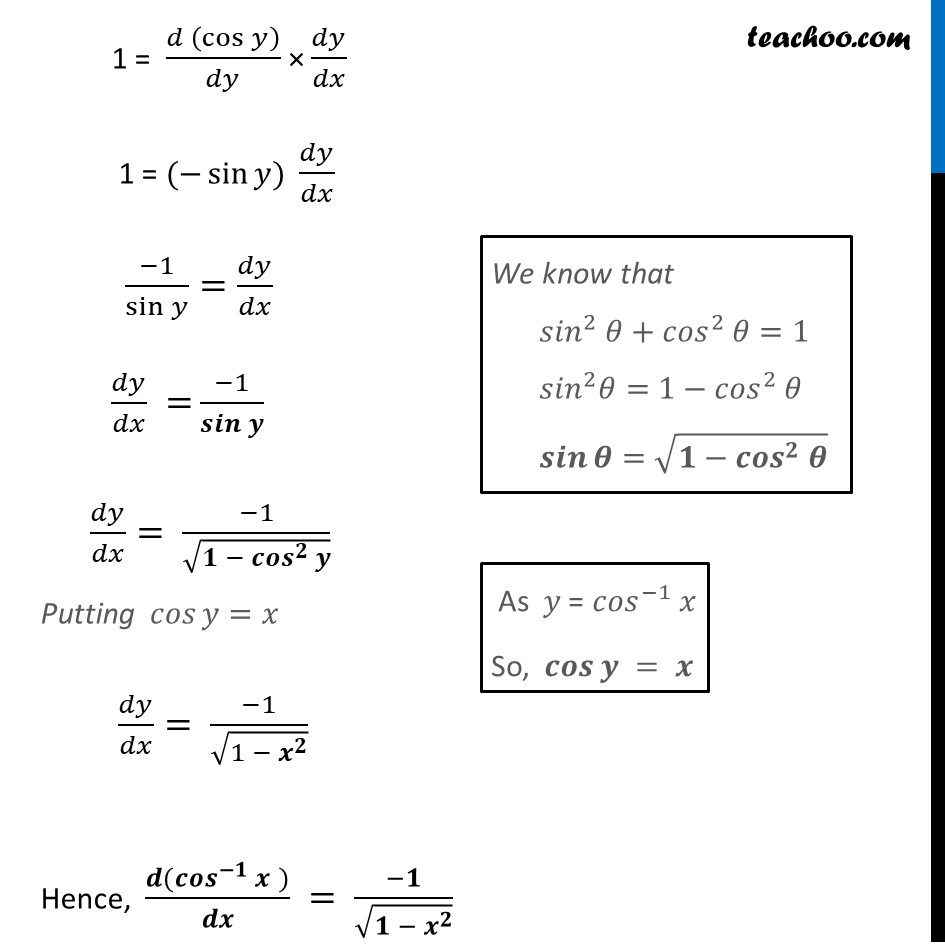Finding derivative of Inverse trigonometric functions

Chapter 5 Class 12 Continuity and Differentiability
Concept wiseLearn in your speed, with individual attention - Teachoo Maths 1-on-1 Class

### Transcript

Derivative of 〖𝒄𝒐𝒔〗^(−𝟏) 𝒙Derivative of 〖𝒄𝒐𝒔〗^(−𝟏) 𝒙 𝑓 (𝑥)=〖𝑐𝑜𝑠〗^(−1) 𝑥 Let 𝒚= 〖𝒄𝒐𝒔〗^(−𝟏) 𝒙 cos⁡〖𝑦=𝑥〗 𝒙=𝐜𝐨𝐬⁡〖𝒚 〗 Differentiating both sides 𝑤.𝑟.𝑡.𝑥 𝑑𝑥/𝑑𝑥 = (𝑑 (cos⁡𝑦 ))/𝑑𝑥 1 = (𝑑 (cos⁡𝑦 ))/𝑑𝑥 × 𝑑𝑦/𝑑𝑦 1 = (𝑑 (cos⁡𝑦 ))/𝑑𝑦 × 𝑑𝑦/𝑑𝑥 1 = (−sin⁡𝑦) 𝑑𝑦/𝑑𝑥 (−1)/sin⁡𝑦 =𝑑𝑦/𝑑𝑥 𝑑𝑦/𝑑𝑥 = (−1)/𝒔𝒊𝒏⁡𝒚 𝑑𝑦/𝑑𝑥= (−1)/√(𝟏 − 〖𝒄𝒐𝒔〗^𝟐 𝒚) Putting 𝑐𝑜𝑠⁡〖𝑦=𝑥〗 𝑑𝑦/𝑑𝑥= (−1)/√(1 − 𝒙^𝟐 ) Hence, (𝒅(〖𝒄𝒐𝒔〗^(−𝟏) 𝒙" " ))/𝒅𝒙 = (−𝟏)/√(𝟏 − 𝒙^𝟐 ) "We know that" 〖𝑠𝑖𝑛〗^2 𝜃+〖𝑐𝑜𝑠〗^2 𝜃=1 〖𝑠𝑖𝑛〗^2 𝜃=1−〖𝑐𝑜𝑠〗^2 𝜃 𝒔𝒊𝒏⁡𝜽=√(𝟏−〖𝒄𝒐𝒔〗^𝟐 𝜽) " " As 𝑦 = 〖𝑐𝑜𝑠〗^(−1) 𝑥 So, 𝒄𝒐𝒔⁡𝒚 = 𝒙